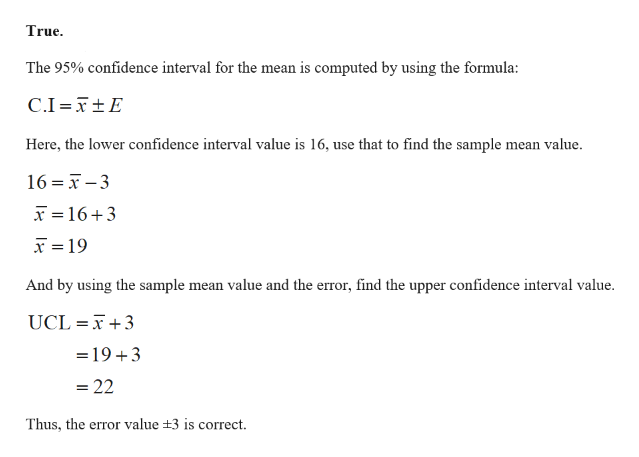# A 95% confidence interval for the mean was computed with a sample of size 90 to be (16,22). Then the Error is ±3.TrueFalse

Question
10 views

A 95% confidence interval for the mean was computed with a sample of size 90 to be (16,22). Then the Error is ±3.

• True
• False
check_circlehelp_outlineImage TranscriptioncloseTrue The 95% confidence interval for the mean is computed by using the formula: C.I TtE Here, the lower confidence interval value is 16, use that to find the sample mean value. 16 -3 16+3 19 And by using the sample mean value and the error, find the upper confidence interval value UCL T3 =19 3 =22 Thus, the error value +3 is correct fullscreen

### Want to see the full answer?

See Solution

#### Want to see this answer and more?

Solutions are written by subject experts who are available 24/7. Questions are typically answered within 1 hour.*

See Solution
*Response times may vary by subject and question.
Tagged in

### Data